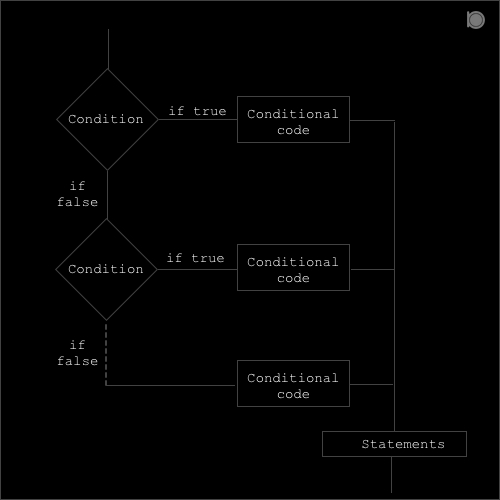if … else if … else statement in C language contains more than two blocks. It provide an optional block for more conditions we want to check for.##### Syntax for if … else if … else statement:
``````if (condition or expression)
{
conditional code;
}
else if (condition or expression)
{
conditional code;
}
.
.
.
.
else
{
condtitonal code;
}
``````

In if … else if … else statement the if block is the true block which means that if the condition is true then the if block is executed but if the condition is false then condition at else if block is checked and if it is true then else if block is executed and so on and finally if all the above conditions are false then the else block is executed.

### Program example of if…else if…else statement in C

``````//An example of if...else if...else statement in C
#include<stdio.h>

int main()
{
int a = 10, b = 20, c = 5;
printf("Consider a= 10, b = 20 and c = 5\n");

if((a>b) && (a>c))
{
printf("a is greater\n");
}
else if ((b>a) && (b>c))
{
printf("b is greater\n");
}
else
{
printf("c is greater\n");
}
printf("This is an example of if...else if...else statement in C\n");
return 0;
}
``````

### Output

``````Consider a= 10, b = 20 and c = 5
b is greater
This is an example of if...else if...else statement in C
``````

In the above example we have three integer variables `a = 10, b = 20 and c = 5`. Here in the if block our condition is `(a>b) && (a>c)` which means that `a` should be greater than both `b` and `c` but it is false because `a` is smaller than `b` and greater than `c` so the if block is skipped and now the condition in else if block is checked. In the else if block we have our condition as `(b>a) && (b>c)` which is true because `b` is greater than both `a` and `c` so the conditional code inside else if block is executed.